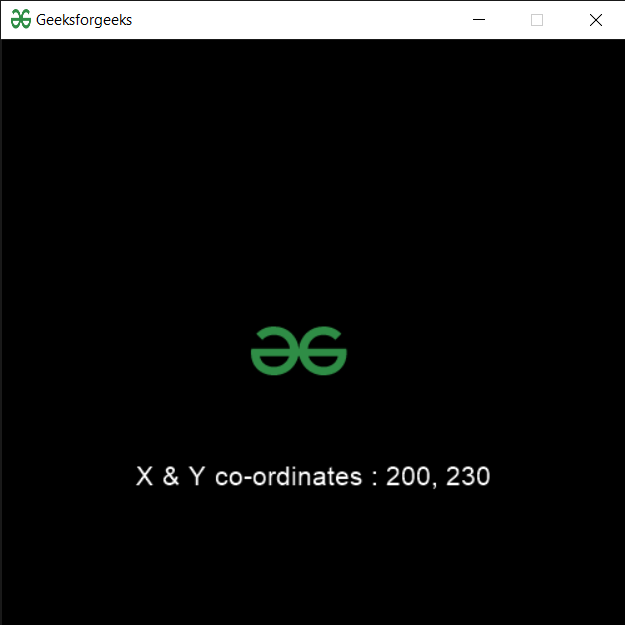GeeksforGeeks App
Open AppBrowser
Continue

# PYGLET – Sprite X and Y Co-ordinates

In this article we will see how we can access X & Y co-ordinates of the sprite in PYGLET module in python. Pyglet is easy to use but powerful library for developing visually rich GUI applications like games, multimedia etc. A window is a “heavyweight” object occupying operating system resources. Windows may appear as floating regions or can be set to fill an entire screen (fullscreen). A sprite is an instance of an image displayed on-screen. Multiple sprites can display the same image at different positions on the screen. Sprites can also be scaled larger or smaller, rotated at any angle and drawn at a fractional opacity. Image is loaded with the help of image module of pyglet. Sprite X & Y co-ordinates are the position of sprite from the x-axis and y-axis.
We can create a window and sprite object with the help of commands given below

```# creating a window
window = pyglet.window.Window(width, height, title)

# creating a sprite object
sprite = pyglet.sprite.Sprite(img, x, y)```

In order to create window we use x and y attribute with sprite object
Syntax : sprite.x and sprite.y
Argument : It takes no argument
Return : It returns integer

Below is the implementation

## Python3

 `# importing pyglet module ``import` `pyglet ``import` `pyglet.window.key as key``   ` `# width of window ``width ``=` `500``   ` `# height of window ``height ``=` `500``   ` `# caption i.e title of the window ``title ``=` `"Geeksforgeeks"``   ` `# creating a window ``window ``=` `pyglet.window.Window(width, height, title) ``   ` `# text  ``text ``=` `"Welcome to GeeksforGeeks"``  ` `# creating label with following properties``# font = cooper``# position = 250, 150``# anchor position = center``label ``=` `pyglet.text.Label(text, ``                          ``font_name ``=``'Cooper'``, ``                          ``font_size ``=` `16``, ``                          ``x ``=` `250``,  ``                          ``y ``=` `150``, ``                          ``anchor_x ``=``'center'``,  ``                          ``anchor_y ``=``'center'``)`` ` ` ` `# creating a batch ``batch ``=` `pyglet.graphics.Batch()`` ` `# loading geeksforgeeks image``image ``=` `pyglet.image.load(``'gfg.png'``)`` ` ` ` ` ` `# creating sprite object``# it is instance of an image displayed on-screen``sprite ``=` `pyglet.sprite.Sprite(image, x ``=` `200``, y ``=` `230``)``   ` `# on draw event ``@window``.event ``def` `on_draw(): ``       ` `    ``# clear the window ``    ``window.clear() ``       ` `    ``# draw the label``    ``label.draw() ``     ` `    ``# draw the image on screen``    ``sprite.draw()``       ` `# key press event     ``@window``.event ``def` `on_key_press(symbol, modifier): ``   ` `    ``# key "C" get press ``    ``if` `symbol ``=``=` `key.C: ``         ` `        ``# printing the message``        ``print``(``"Key : C is pressed"``)``         ` `# image for icon ``img ``=` `image ``=` `pyglet.resource.image(``"gfg.png"``) `` ` `# setting image as icon ``window.set_icon(img) `` ` `# accessing x, y co-ordinates of the sprite``value_x ``=` `sprite.x``value_y ``=` `sprite.y`` ` `# creating text from value``text ``=` `"X & Y co-ordinates : "` `+` `str``(value_x) ``+` `", "` `+` `str``(value_y)`` ` `# setting text to the label``label.text ``=` `text``    ` `# start running the application ``pyglet.app.run()`

Output :My Personal Notes arrow_drop_up